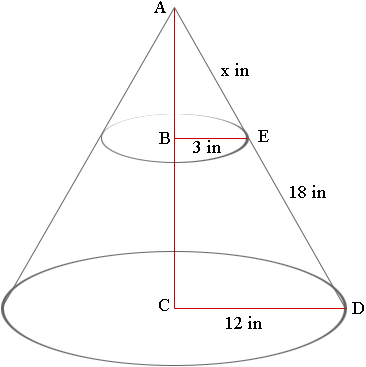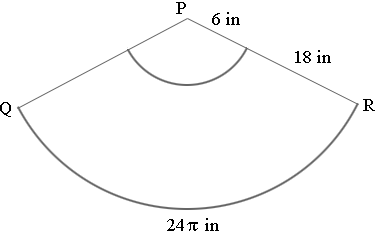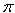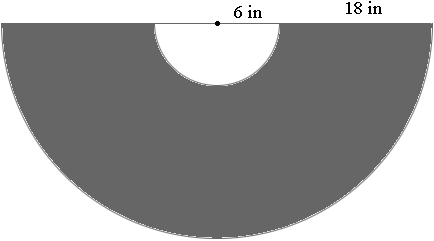SEARCH HOMEMath Central Quandaries & QueriesQuestion from Arnold: I am making a metal shroud for a outdoor fireplace, it is basically a lampshade type pattern,like the bottom of a cone.The top has to be 6 inches to fit the 6 inch stovepipe,and the bottom will be a 24 inch circle. the sides will be 18 inches in length.With the cost of the sheet metal,I can only afford to cut this out once,can you help me with the pattern?Arnold,

I drew an image of your shroud, extended it to the apex at the top and labeled some points.I want to determine the distance from A to E, what I have called x inches in the diagram. Triangles ACD and ABE are similar so

x/3 = (18+x)/12

Solving this equation for x gives x = 6 inches. Thus your pattern will be cut from a sector of a circle of radius 18 + 6 = 24 inches.The length of the arc QR is the circumference of the circle at the base of the cone in the first diagram. The circumference of a circle is 2r and the radius of the circle at the base of the cone is 12 inches so the length of the arc QR is 24inches.

This arc is a portion of the circumference of the circle with centre P and radius 24 inches. The circumference of this circle is 2r which is 48inches. Hence the arc QR is half circumference of the circle and thus the angle RPQ is 180 degrees. So, here is the patternCut out a semicircle of radius 24 inches and then remove a concentric circle of radius 6 inches.

If you have access to a digital camera, send us a photo of the completed project.
PennyMath Central is supported by the University of Regina and The Pacific Institute for the Mathematical Sciences.